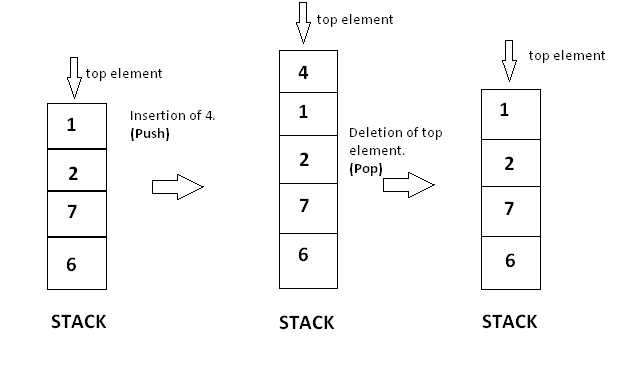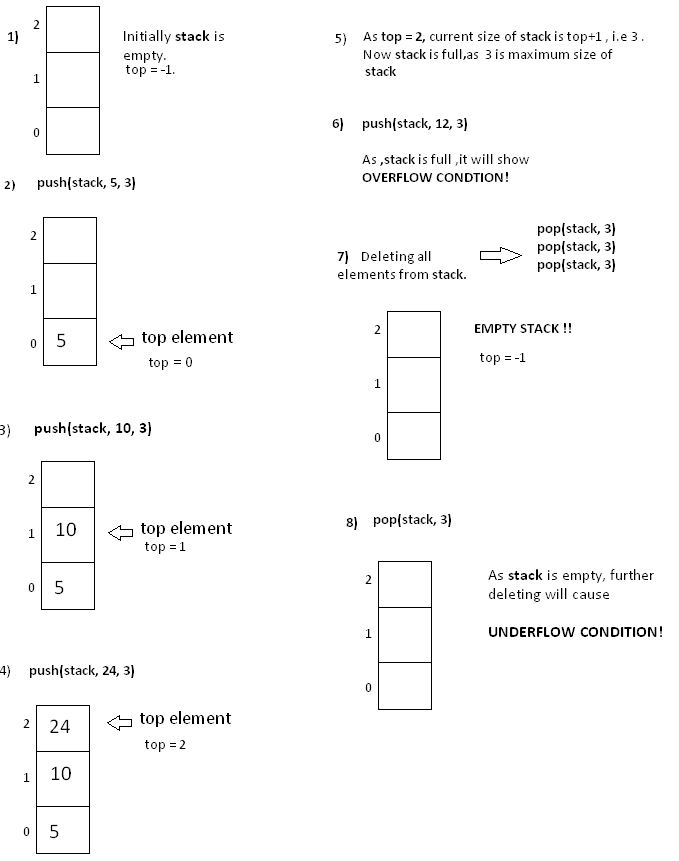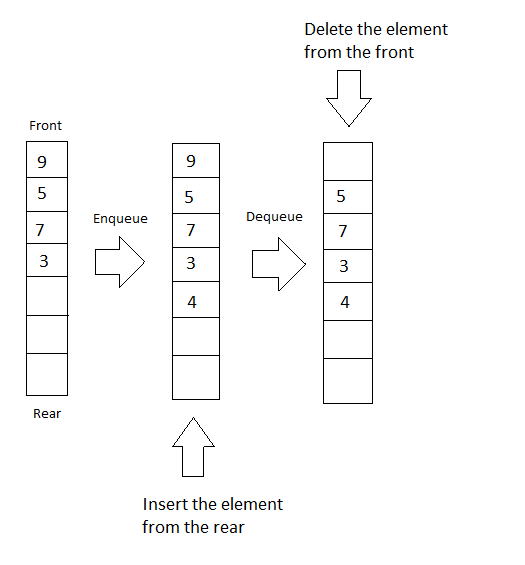119
Stacks And Queues
Stack
Queue
CodeMonk

We deal with data all the time, so how we store, organise or group our data, matters.

Data Structures are tools which are used to store data in a structured way in computer to use it efficiently. Efficient data structures plays a vital role in designing good algorithms. Data structures are considered as key organising factors in software design in some designing methods and programming languages. Examples: In english dictionaries, we can access any word easily as the data is stored in a sorted way using a particular data structure.

In online city map, data like position landmark, road network connections, we show this data using geometry using two dimensional plane.

Here, we will discuss about Stacks and Queues Data Structures.

Stacks: Stack is collection of elements, that follows the LIFO order. LIFO stands for Last In First Out, which means element which is inserted most recently will be removed first. Imagine a stack of tray on the table. When you put a tray there you put it at top, and when you remove it, you also remove it from top.

A stack has a restriction that insertion and deletion of element can only be done from only one end of stack and we call that position as top. The element at top position is called top element. Insertion of element is called PUSH and deletion is called POP.Operations on Stack:

push( x ) : insert element x at the top of stack.

void push (int stack[ ] , int x , int n) {
if ( top == n-1 ) {         //if top position is the last of position of stack, means stack is full .
cout << “Stack is full.Overflow condition!” ;
}
else{
top = top +1 ;            //incrementing top position
stack[ top ] = x ;       //inserting element on incremented position .
}
}


pop( ) : removes element from the top of stack.

void pop (int stack[ ] ,int n )
{

if( isEmpty ( ) )
{
cout << “Stack is empty . Underflow condition! ” << endl ;
}
else
{
top = top - 1 ; //decrementing top’s position will detach last element from stack .
}
}


topElement ( ) : access the top element of stack.

int topElement ( )
{
return stack[ top ];
}


isEmpty ( ) : check whether the stack is empty or not.

bool isEmpty ( )
{
if ( top == -1 )  //stack is empty .
return true ;
else
return false;
}


size ( ) : tells the current size of stack .

int size ( )
{
}


Implementation:

#include <iostream>
using namespace std;
int top = -1; //globally defining the value of top ,as the stack is empty .

void push (int stack[ ] , int x , int n)
{
if ( top == n-1 )          //if top position is the last of position of stack, means stack is full .
{
cout << “Stack is full.Overflow condition!” ;
}
else
{
top = top +1 ;            //incrementing top position
stack[ top ] = x ;       //inserting element on incremented position .
}
}
bool isEmpty ( )
{
if ( top == -1 )  //stack is empty .
return true ;
else
return false;
}
void pop (int stack[ ] ,int n )
{

if( isEmpty ( ) )
{
cout << “Stack is empty . Underflow condition! ” << endl ;
}
else
{
top = top - 1 ; //decrementing top’s position will detach last element from stack .
}
}
int size ( )
{
}
int topElement ( )
{
return stack[ top ];
}
// Now lets implementing these functions on the above stack

int main( )
{
int stack[ 3 ];
// pushing element 5 in the stack .
push(stack , 5 , 3 ) ;

cout << “Current size of stack is ” << size ( ) << endl ;

push(stack , 10 , 3);
push (stack , 24 , 3) ;

cout << “Current size of stack is ” << size( ) << endl ;

//As now the stack is full ,further pushing  will show overflow condition .
push(stack , 12 , 3) ;

//Accessing the top element .
cout << ”The current top element in stack is “ << topElement( ) << endl;

//now removing all the elements from stack .
for(int i = 0 ; i < 3;i++ )
pop( );
cout << “Current size of stack is ” << size( ) << endl ;

//as stack is empty , now further popping will show underflow condition .
pop ( );

}


Output :
Current size of stack is 1
Current size of stack is 3
The current top element in stack is 24
Stack is full.Overflow condition!
Current size of stack is 0
Stack is empty . Underflow condition!

It will be more clear with the pictorial representation of operations performed in the code.Balanced parenthesis problem : You have a string that contains a mathematical equation where you have to check, whether the given string has balanced parentheses or not. Balanced parenthesis means that the given string should have equal number of opening and closing parenthesis.

We can check this using stack. Lets see how.

We can maintain a stack where we store a parenthesis, whenever we encounter an opening parenthesis in the string (can push in stack )and pop a parenthesis from the stack whenever we encounter a closing parenthesis.

#include <iostream>
using namespace std;
int top;
void  check (char str[ ], int n, char stack [ ])
{
for(int i = 0 ; i < n ; i++ )
{
if (str [ i ] == ‘(’)
{
top = top + 1;
stack[ top ] = ‘ ( ’;
}
if(str[ i ] == ‘)’ )
{
if(top == -1 )
{
top = top -1 ;
break ;
}
else
{
top = top -1 ;
}
}
}
if(top == -1)
cout << “String is balanced!” << endl;
else
cout << “String is unbalanced!” << endl ;
}

int main ( )
{
//balanced parenthesis string.
char str[  ] = { ‘(‘ , ‘a’ , ‘+’, ‘ ( ’, ‘b ’ , ‘-’ , ‘ c’ ,‘)’ , ‘ ) ’} ;

// unbalanced string .
char str1 [ ] = { ‘(’ , ‘(’ , ‘a’ , ‘ + ’ , ‘ b’ , ‘)’ } ;
char stack [ 15 ] ;
top = -1;
check (str , 9 , stack );      //passing balanced string
top = -1 ;
check(str1 , 5 , stack) ;    //passing unbalanced string
return 0;

}


Output :
String is balanced!
String is unbalanced!

Here are the C++ STL tools for Stacks which are in "stack" header file.

Declaration:

stack <int> s;


Push:

s.push(element);


Pop:

s.pop();


Top Element:

s.top()


IsEmpty:

s.empty()


Size:

s.size()


Implementation of STL Functions:

#include <iostream>
#include <stack>
using namespace std;
int main( )
{
stack <int> s;  // declaration of stack

//inserting 5 elements in stack from 0 to 4.
for(int i = 0;i < 5; i++)
{
s.push( i ) ;
}

//Now the stack is {0, 1, 2, 3, 4}

//size of stack s
cout<<”Size of stack is: ” <<s.size( )<<endl;

//accessing top element from stack, it will be the last inserted element.
cout<<”Top element of stack is: ” <<s.top( ) <<endl ;

//Now deleting all elements from stack
for(int i = 0;i < 5;i++)
{
s.pop( );
}

//Now stack is empty,so empty( ) function will return true.

if(s.empty())
{
cout <<”Stack is empty.”<<endl;
}
else
{
cout <<”Stack is Not empty.”<<endl;
}

return 0;

}


Output:
Size of stack is: 5
Top element of stack is: 4
Stack is empty.

Queue:
Queue is a data structure that follows the FIFO principle. FIFO means First In First Out i.e the element added first in the queue will be the one to be removed first. Elements are always added to the back and removed from the front. Think of it as a line of people waiting for a bus at the bus stand. The person who will come first will be the first one to enter the bus.

Queue supports some fundamental functions:

Enqueue: Adds an element to the back of the queue if the queue is not full otherwise it will print “OverFlow”.

void enqueue(int queue[], int element, int& rear, int arraySize) {
if(rear == arraySize)            // Queue is Full
printf(“OverFlow\n”);
else {
queue[rear] = element;    // Add the element to the back
rear++;
}
}


Dequeue: Removes the element from the front of the queue if the queue is not empty otherwise it will print “UnderFlow”.

void dequeue(int queue[], int& front, int rear) {
if(front == rear)            // Queue is empty
printf(“UnderFlow\n”);
else {
queue[front] = 0;        // Delete the front element
front++;
}
}


Front: Return the front element of the queue

int Front(int queue[], int front) {
return queue[front];
}


There are some support functions also:

Size: Returns the size of the queue or the number of elements in the queue.

int size(int front, int rear) {
return (rear - front);
}


IsEmpty: Returns true if the queue is empty otherwise returns false.

bool isEmpty(int front, int rear) {
return (front == rear)
}Let us try a problem.

We are given a string and we have to perform some steps. In each step we need to take the first character of the string and put it at the end of the string. We have to find out what will be the string after N steps.

Clearly we can consider the string as a queue. At each step we just dequeue the character from the front and enqueue it at the end and repeat the process N times. Let us code it.

#include <iostream>
#include <cstdio>

using namespace std;

void enqueue(char queue[], char element, int& rear, int arraySize) {
if(rear == arraySize)            // Queue is Full
printf("OverFlow\n");
else {
queue[rear] = element;    // Add the element to the back
rear++;
}
}

void dequeue(char queue[], int& front, int rear) {
if(front == rear)            // Queue is empty
printf("UnderFlow\n");
else {
queue[front] = 0;        // Delete the front element
front++;
}
}

char Front(char queue[], int front) {
return queue[front];
}

int main() {
char queue = {'a', 'b', 'c', 'd'};
int front = 0, rear = 4;
int arraySize = 20;                // Size of the array
int N = 3;                    // Number of steps
char ch;
for(int i = 0;i < N;++i) {
ch = Front(queue, front);
enqueue(queue, ch, rear, arraySize);
dequeue(queue, front, rear);
}
for(int i = front;i <= rear;++i)
printf("%c", queue[i]);
printf("\n");
return 0;
}


Output:
dabc

C++ provides built-in tools to create and use queues. These tools are in Standard Template Library "queue" header file. You can use these tools after you’ll get comfortable with the functioning of queues.

Declaration:

queue <int> q;


Enqueue:

q.push(element);


Dequeue:

q.pop();


Front:

q.front()


IsEmpty:

q.empty()


Size:

q.size()


Now try to solve the above example using STL.

#include <iostream>
#include <cstdio>
#include <queue>

using namespace std;

int main() {
char qu = {'a', 'b', 'c', 'd'};
queue <char> q;
int N = 3;                            // Number of steps
char ch;
for(int i = 0;i < 4;++i)
q.push(qu[i]);
for(int i = 0;i < N;++i) {
ch = q.front();
q.push(ch);
q.pop();
}
while(!q.empty()) {
printf("%c", q.front());
q.pop();
}
printf("\n");
return 0;
}


Output:
dabc